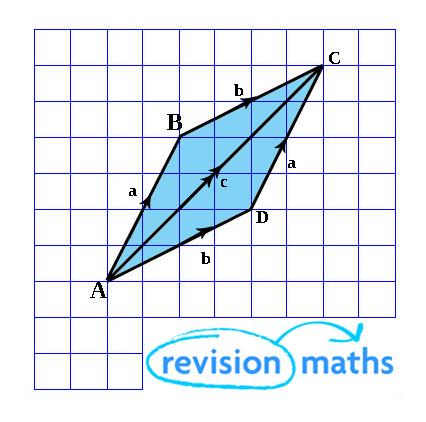## Vectors

Calculating the Modulus of a Vector
In this section, you will learn how to calculate the modulus of a vector. The modulus is a mathematical term for the length or the magnitude.

This video and text below takes a look at Vectors and Scalars.

The magnitude of vector x is written as |x|.

The magnitude of vector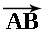is written as |AB|.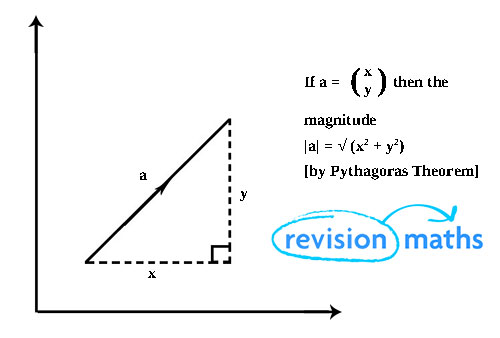Zero vector and unit vectors

A vector with magnitude 0 is called the zero vector, written 0. A vector with magnitude 1 is called a unit vector.

Vectors are equal if they have the same magnitude and the same direction.

a = b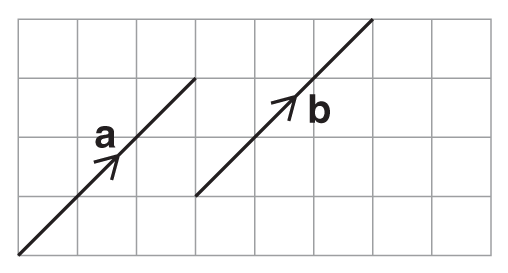Inverse Vectors

The inverse of a vector is a vector of equal magnitude but in the opposite direction. The inverse of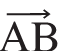is -or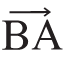and the inverse of a is -a.

Scalars

Scalars have magnitude but not direction. Vectors can be multiplied by a scalar to produce another vector.

Multiplying vector x by 3 will give a new vector 3 times the length and parallel to x.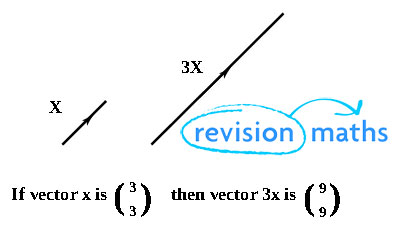When 2 vectors are added or subtracted the vector produced is called the resultant.

The resultant is identified by a double arrowhead.

Triangle Law:

To add two vectors you apply the first vector and then the second.+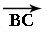=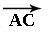or

a + b = c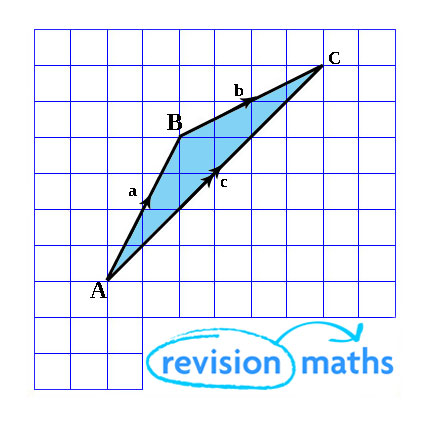Subtracting a vector is the same as adding its inverse.

a – b is the same as a + (-b)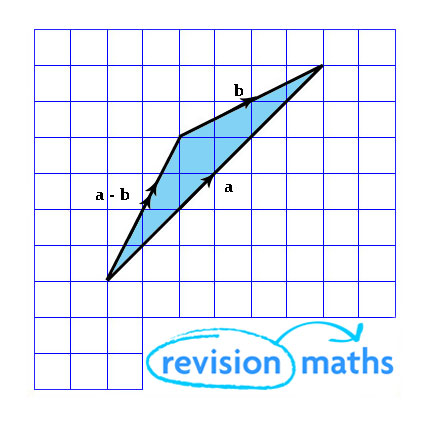Parallelogram Law:

Moving from A to C through B is the same as moving through D.+=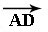+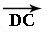=or

a + b = b + a = c